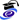Luca Di PersioPosition
Temporary Assistant Professor
MAT/06 - PROBABILITY AND STATISTICS
Research sector (ERC)
PE1_13 - Probability

Office
Ca' Vignal 2,  Floor 2,  Room 10.A
Telephone
+39 045 802 7968
Fax
+39 045 802 7068
E-mail
lucadipersiounivritCurriculum
•Curriculum Vitae (Eng)   (pdf, en, 1159 KB, 21/10/17)
•Curriculum Vitae (It)   (pdf, it, 1213 KB, 21/10/17)

The research activity of Luca Di Persio is mainly focused on the following topics:
•  Stochastic Partial Differential Equations (SPDEs) both in finite and infinite dimensions
• Asymptotic Expansion of finite-infinite Integrals
•  Interacting Particle Systems, Random Walk in Random Media
•  Mean Field Games
•  Time series Analysis and applications to Mathematical Finance
• Numerical Methods for Mathematical Finance
• Neural networks and applications

His articles are published by journals mainly devoted to Stochastic Analysis and its applications, particularly with respect to the modelisation of problems arising in the context of Mathematical Finance. Whitin the same ambit takes place his congressual activity, both as organizer and invited speaker to internationally recognized consesses.

His didactic activity, which extends to the organization of courses and lectures within the Stochastic Analysis and applications framework, is mainly devoted to the Theory of Probability and time series analysis. In particular, Luca Di Persio is in charge for the didactic path in Mathematical Finance (Master degree in Mathematics) and he teaches, or  he has taugtht, the following courses: Probability, Stochastic Systems, Stochastic Differential Euqations, Mathematical Finance.

Luca Di Persio is also
• member of the PhD School in Mathematics, jointly organised by the Mathematics Department of the University of Trento and by the College of Mathematics of the Computer Science Department of the University of Verona
• coordinator for the European mobility program Erasmus+, activated with the universities of Bielefeld, Munich, Oslo and Wuppertal.

Modules

Modules running in the period selected: 25.
Click on the module to see the timetable and course details.

Course Name Total credits Online Teacher credits Modules offered by this teacher
Master's degree in Mathematics Mathematical finance (2019/2020)   6Master's degree in Mathematics Stochastic Calculus (2019/2020)   6
Bachelor's degree in Applied Mathematics Stochastic systems (2019/2020)   6Master's degree in Mathematics Mathematical finance (2018/2019)   6(Parte 1)
(Parte 2)
Master's degree in Mathematics Stochastic differential equations (2018/2019)   6Bachelor's degree in Applied Mathematics Stochastic systems (2018/2019)   6(Teoria)
Master's degree in Mathematics Mathematical finance (2017/2018)   6Master's degree in Mathematics Stochastic differential equations (2017/2018)   6Bachelor's degree in Applied Mathematics Stochastic systems (2017/2018)   6Master's degree in Mathematics Mathematical finance (2016/2017)   6Bachelor's degree in Applied Mathematics Stochastic systems (2016/2017)   6
Master's degree in Mathematics Mathematical finance (2015/2016)   6(Teoria 1)
Bachelor's degree in Applied Mathematics Stochastic systems (2015/2016)   6    (Analisi di serie temporali)
(Catene di Markov in tempo discreto)
Master's degree in Mathematics Mathematical finance (2014/2015)   6    (Teoria 1)
(Esercitazioni)
Bachelor's degree in Applied Mathematics Probability (2014/2015)   6    (Teoria)
Master's degree in Mathematics Mathematical finance (2013/2014)   6    (Teoria 1)
(Esercitazioni)
Bachelor's degree in Applied Mathematics Probability (2013/2014)   6(Teoria)
Bachelor's degree in Applied Mathematics Probability (2012/2013)   6    (Teoria)
(Esercitazioni)
Bachelor's degree in Applied Mathematics Probability (2011/2012)   6
Bachelor's degree in Computer Science Probability and Statistics (2011/2012)   6    (Teoria)